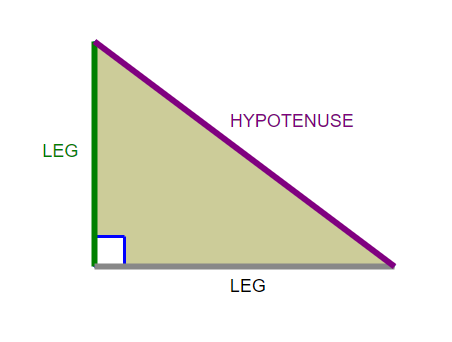﻿ The Pythagorean Theorem

#The Pythagorean Theorem

(This lesson also appears in the Algebra I curriculum. If you studied it there, then just quickly review, and move on to the next section!)

A 90° angle is called a right angle. A right triangle is a triangle with a 90° angle.

In a right triangle, the side opposite the $\,90^\circ$ angle is called the hypotenuse and the remaining two sides are called the legs.

The angles in any triangle add up to $\,180^\circ\,.$In any triangle, the longest side is opposite the largest angle, and the shortest side is opposite the smallest angle. Thus, in a right triangle, the hypotenuse is always the longest side.

The Pythagorean Theorem gives a beautiful relationship between the lengths of the sides in a right triangle: the sum of the squares of the shorter sides is equal to the square of the hypotenuse. Furthermore, if a triangle has this kind of relationship between the lengths of its sides, then it must be a right triangle!

The Pythagorean Theorem
Let $\,\,T\,\,$ be a triangle with sides of lengths $\,a\,,$ $\,b\,,$ and $\,c\,,$ where $\,c\,$ is the longest side (if there is a longest side). Then: $$\begin{gather} \cssId{s21}{\text{T\, is a right triangle}}\cr \cssId{s22}{\text{if and only if}}\cr \cssId{s23}{a^2 + b^2 = c^2} \end{gather}$$Have fun with many proofs of the Pythagorean Theorem! This applet (you'll need Java) is one of my favorites. Let it load, then keep pressing ‘Next’.

## Examples

Question: Suppose that two angles in a triangle are $\,60^\circ$ and $\,30^\circ.$ Is it a right triangle? Answer YES, NO, or MAYBE.
Solution: Yes. The third angle must be $\,180^\circ - 60^\circ - 30^\circ = 90^\circ\,.$
Question: Suppose that a triangle has a $\,100^\circ$ angle. Is it a right triangle? Answer YES, NO, or MAYBE.
Solution: No. The remaining two angles must sum to $\,80^\circ,$ so neither remaining angle is a $\,90^\circ$ angle.
Question: Suppose that a triangle has a $\,70^\circ$ angle. Is it a right triangle? Answer YES, NO, or MAYBE.
Solution: Maybe. The remaining two angles must sum to $\,110^\circ,$ so one of the remaining angles could be a $\,90^\circ$ angle.
Question: Suppose the legs of a right triangle have lengths $\,3\,$ and $\,x\,,$ and the hypotenuse has length $\,5\,.$ Find $\,x\,.$
Solution: $$\begin{gather} \cssId{s53}{3^2 + x^2 = 5^2}\cr \cssId{s54}{9 + x^2 = 25}\cr \cssId{s55}{x^2 = 16}\cr \cssId{s56}{x = 4} \end{gather}$$

Note:  $x\,$ cannot equal $\,-4\,,$ because lengths are always positive.

The $\,3{-}4{-}5\,$ triangle is a well-known right triangle. Multiplying all the sides of a triangle by the same positive number does not change the angles. Thus, if you multiply the sides of a $\,3{-}4{-}5\,$ triangle by any positive real number $\,k\,,$ then you will still have a right triangle. For example, these are all right triangles:

 $6{-}8{-}10$ ($\,k = 2\,$) $9{-}12{-}15$ ($\,k = 3\,$) $1.5{-}2{-}2.5$ ( $\,k = 0.5\,$ ) $3\pi{-}4\pi{-}5\pi$ ($\,k = \pi\,$ ) and so on!
Question: Suppose a triangle has sides of lengths $\,1\,,$ $\,\sqrt{3}\,,$ and $\,2\,.$   Is it a right triangle?
Solution: Yes. Since $\,2 \gt \sqrt{3}\,,$ the longest side has length $\,2\,.$ And: $$\cssId{s81}{1^2 + {(\sqrt{3})}^2} \cssId{s82}{= 1 + 3} \cssId{s83}{= 4} \cssId{s84}{= 2^2}$$